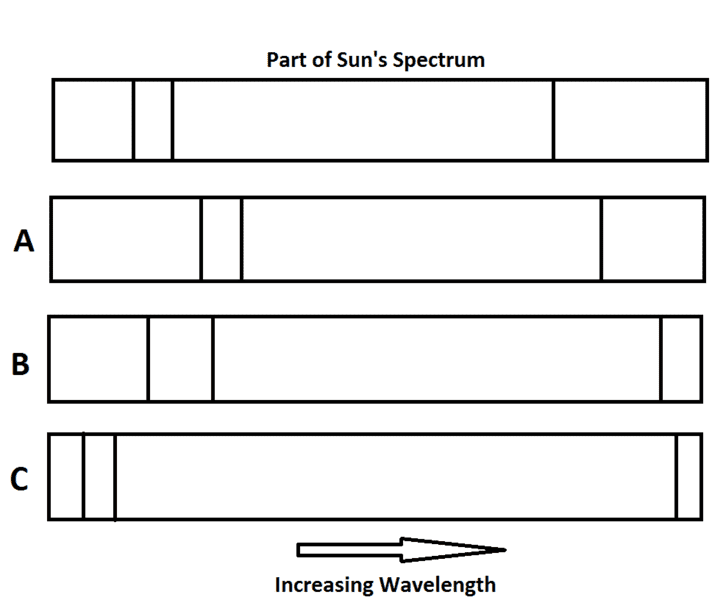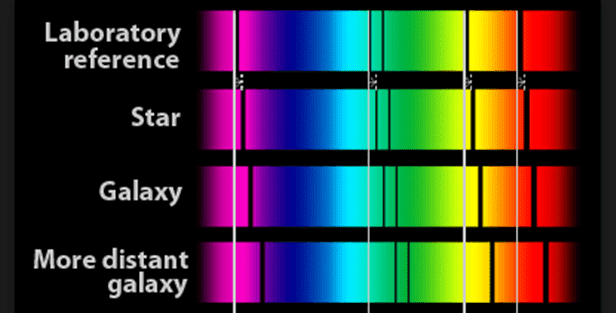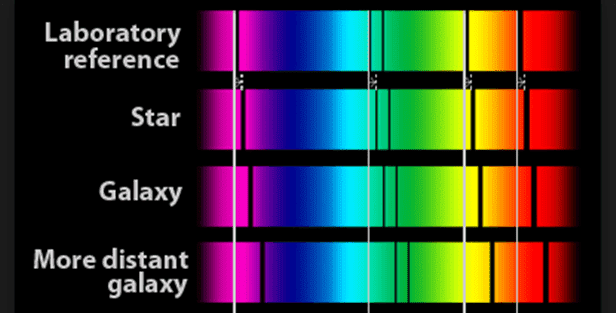# Emission Spectra of Stars

## Homework Statement

[/B]
The top spectrum shows part of the Sun's spectrum. State and explain which letter best represents the light from a star that is similar to the Sun.None.

## The Attempt at a Solution

I originally was thinking A but my book says B but only gives numerical answers and no explanation. Even though it mentions nothing about red shift in the question I am thinking it must be implying that this star is showing red shift? All the lines in A have shifted by the same amount and therefore I thought they would be giving off light the same as the Sun. The only guess at why B might be correct was that I was thinking that bigger wavelengths will shift more than shorter wavelengths (this is a logical guess that may be wrong). I thought about it like this. If I give the first line for the Sun a number like 1, then second line 2 and the last line 8. If the space between the Sun and this star doubled for example whilst the light was travelling to us then the wavelength of the first line will be twice as big, the second line 4 times and the last line 16 times. Again, I have no idea if this actually happens as all diagram on google images do not show an increase in the distance between adjacent spectral lines. I am just trying to come up with an idea to fit the answer.

Thanks for any help given.

## Answers and Replies

BvU
Science Advisor
Homework Helper
this is a logical guess that may be wrong
That's where your relevant equation (oops, misssing?) should help you out. Work it out semi quantitatively and you will be able to decide for yourself !

And: any star similar to the sun should be further away than the sun, right ?

That's where your relevant equation (oops, misssing?) should help you out. Work it out semi quantitatively and you will be able to decide for yourself !

And: any star similar to the sun should be further away than the sun, right ?

This equation is the only one we have used:

Δλ/λ = v/c

In this example, Δλ = (λstar - λsun)

So, λstar = vλsun/c + λsun

So according to this (I think), as you consider larger wavelengths from the Sun, the wavelengths of the star will shift by a bigger factor? So my qualitative answer in my OP was also right?

Thanks

BvU
Science Advisor
Homework Helper
This equation is the only one we have used
And it's the right equation too ! In A you see the long wavelength spectral line move less than the short length ones. In B it looks a bit more in proportion.

•Jimmy87
In A you see the long wavelength spectral line move less than the short length ones

Thanks. That is my bad drawing of the textbook question - all the lines in A shift to the right by the same amount. So after what we discussed this diagram I found on the internet must be wrong:In this diagram the original spacing between spectral lines in the reference frame is maintained but if I am understanding things correctly the spacing must increase because of the " + λreference" term on the end which makes longer wavelengths shift more than shorter ones. Is that right?

#### Attachments

BvU
Science Advisor
Homework Helper
Ah, but where is ##\lambda = 0## ?

Ah, but where is ##\lambda = 0## ?
It doesn't does it? So does the spacing between adjacent spectral lines increase or not? Otherwise the answer to the OP is wrong.

BvU
Science Advisor
Homework Helper
It doesn't does it?
Funny answer to the question 'where is ##\lambda = 0 ##?'You'd have to do some math to claim the picture in the OP is utterly wrong, but even then A is more wrong than B.

By teh way:
##\lambda = 0 ## is far away to the left in the book and in the post #1 picture.

Picture borrowed from https://www.quora.com/Whats-the-range-of-wavelengths-of-visible-light-from-red-to-violetFunny answer to the question 'where is ##\lambda = 0 ##?'You'd have to do some math to claim the picture in the OP is utterly wrong, but even then A is more wrong than B.

By teh way:
##\lambda = 0 ## is far away to the left in the book and in the post #1 picture.

Picture borrowed from https://www.quora.com/Whats-the-range-of-wavelengths-of-visible-light-from-red-to-violetI take it you mean Δλ is zero? So yes I can see that would be the case in the lab reference frame. Why would picture B be technically wrong. I thought the equation is really clear. The bigger the wavelength, the bigger the shift - i.e. spacing between adjacent spectral lines increases.

SammyS
Staff Emeritus
Science Advisor
Homework Helper
Gold Member
I take it you mean Δλ is zero? So yes I can see that would be the case in the lab reference frame. Why would picture B be technically wrong. I thought the equation is really clear. The bigger the wavelength, the bigger the shift - i.e. spacing between adjacent spectral lines increases
I suspect that the wavelength scale is logarithmic in your figure from post #5 (repeated below.).That would make shift for the lines appear to be the same for a given star or galaxy.

Of course it can be argued that the wavelength scale in the original post could be logarithmic, making A the correct response.

#### Attachments

Last edited:
•Jimmy87
BvU
Science Advisor
Homework Helper
Of course it can be argued that the wavelength scale in the original post could be logarithmic, making A the correct response
No.